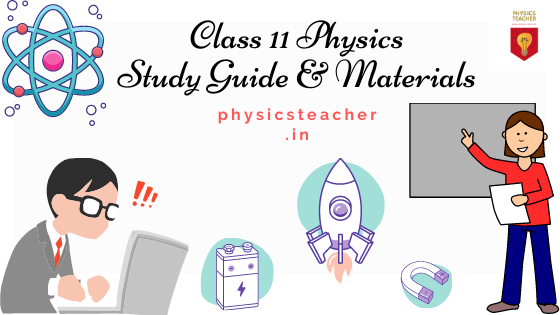# 11th Physics — how to study [class 11 physics Guide & Study Materials]

This post is to guide the students who have opted for Physics as a subject for their class 11. We will have a broad view of the topics and chapters to be studied and also refer to the books and online study materials as a quick reckoner for the 11th Physics study, covering the NCERT syllabus. Hopefully, students will find it useful to start the ball rolling and as well as, plan the study well ahead with confidence. [ Read the complete post: here ]11th Physics — how to study [Physics study guide class 11 & study notes & materials]

# 11th Physics — how to study [Physics study guide class 11 & study notes & materials]

Don’t get nervous seeing the chapters to cover. If you focus on concept building from the very beginning, your journey will be more enjoyable.

In the following sections, we will try to find out how to go ahead with a steady plan and where to get the study materials for a better understanding of the topics.

# How to study

• And, get ready for the next chapters.

# How to study

• Significant figures — this is important to go through and the rules should be noted down and followed while solving numerical problems.
• Rest of the topics — go through for an all-round understanding of the units and measurements of the physical quantities.
[ Read the complete post: here ]

# How to study

• The derivations, graphs, and numerical are to be done properly.

[ Read the complete post: here ]

# Online study material & notes [class 11 chapter 3 — Motion in a straight line]

Instantaneous Velocity — definition & equation with solved problem

Average velocity — definition, formula

Difference between Instantaneous Speed and Instantaneous Velocity

Difference between average speed and average velocity

Instantaneous Acceleration — definition & formula with solved problem

Average Acceleration and its formula & solved numerical problems

Derivation of Motion Equations (SUVAT equations)

How to draw motion graphs on a graph paper

Velocity time graph

Distance time graph

# Online study material & notes [class 11 chapter 4 — Motion in a plane]

Vector Subtraction

Vector Product | Vector Multiplication

Vector Resolution

Vector — Numerical worksheet

Scalar product & Vector product examples

Projectile Motion — derivation & other important FAQs

Projectile — velocity, acceleration & displacement at different points

Projectile formula listing

Uniform Circular motion & acceleration

Relationship between circular motion and linear motion physical quantities

# How to study

• Study the …[ Read the complete post: here ]

# How to study

This chapter brings in the topics related to the rotational domain of motion and hence the relationships with some important quantities of rotational domain with its corresponding counterpart in linear motion are to be studied. This will help to build up a better understanding of rotational motion.

# How to study

• Three Laws of Kepler — (a) Law of orbits (b) Law of areas, & © the Law of Periods are to be studied
• Newton’s Universal [ Read the complete post: here ]

# Online study material & notes [class 11 chapter 8 — Gravitation]

Here, we have covered 8 chapters for the class 11 study. We are coming again to update this topic with the details for the rest of the chapters.

11th Physics — how to study [class 11 physics Guide & Study Materials]

Originally published at https://physicsteacher.in on May 12, 2021.

NIT Grad & IT prof. Writes and teaches HS physics. A motivational speaker and an active blogger with multiple niches. With lots of stories to tell.

## More from Anupam

NIT Grad & IT prof. Writes and teaches HS physics. A motivational speaker and an active blogger with multiple niches. With lots of stories to tell.